# `20180721a` - Testing to format the data for experiments to be easily reproduced

## Objectives

The objective of this experiment is to check :

1. If class-oriented library works
2. The TGC functionning, and its calibration
3. Have fun introducing new class functions

## Results

### Type of signal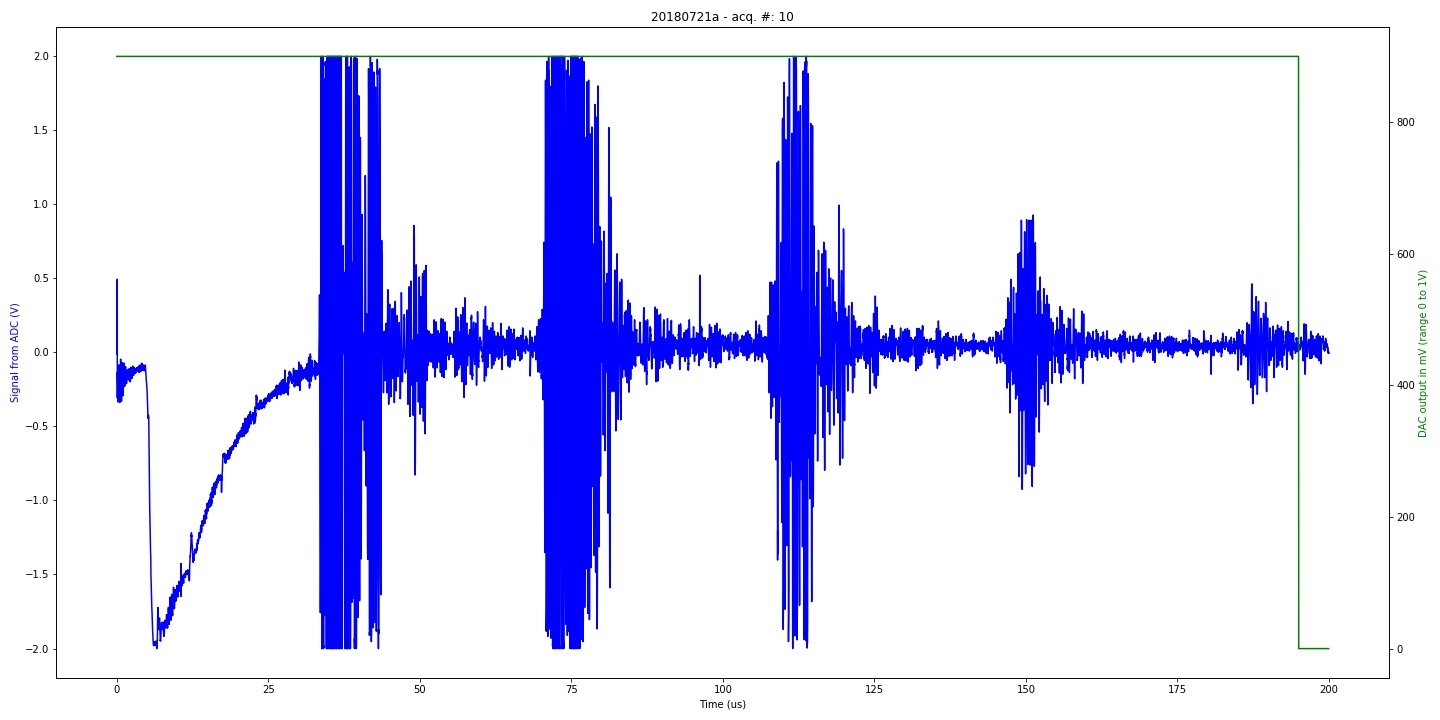### Curve of the gain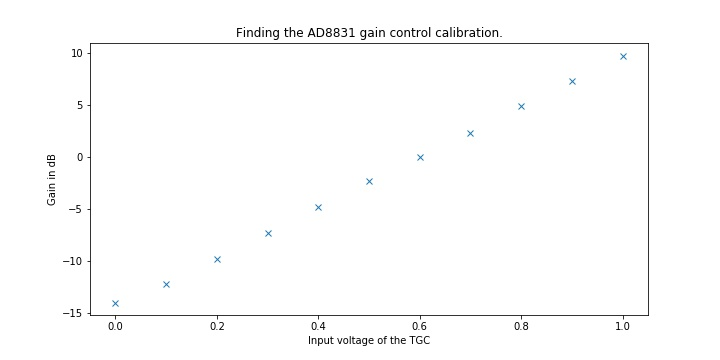### Impact of gain on noise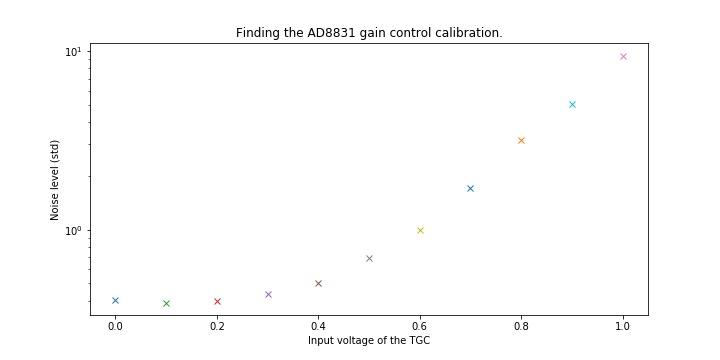### FFT - content of such a line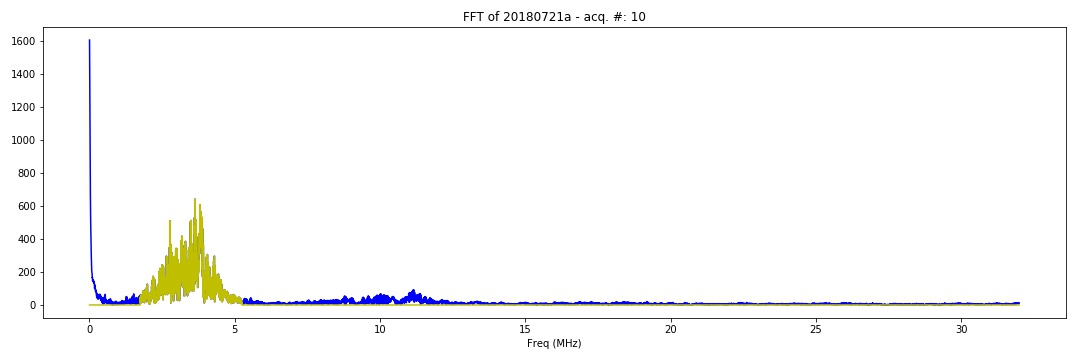# Images of the Experiment

## Others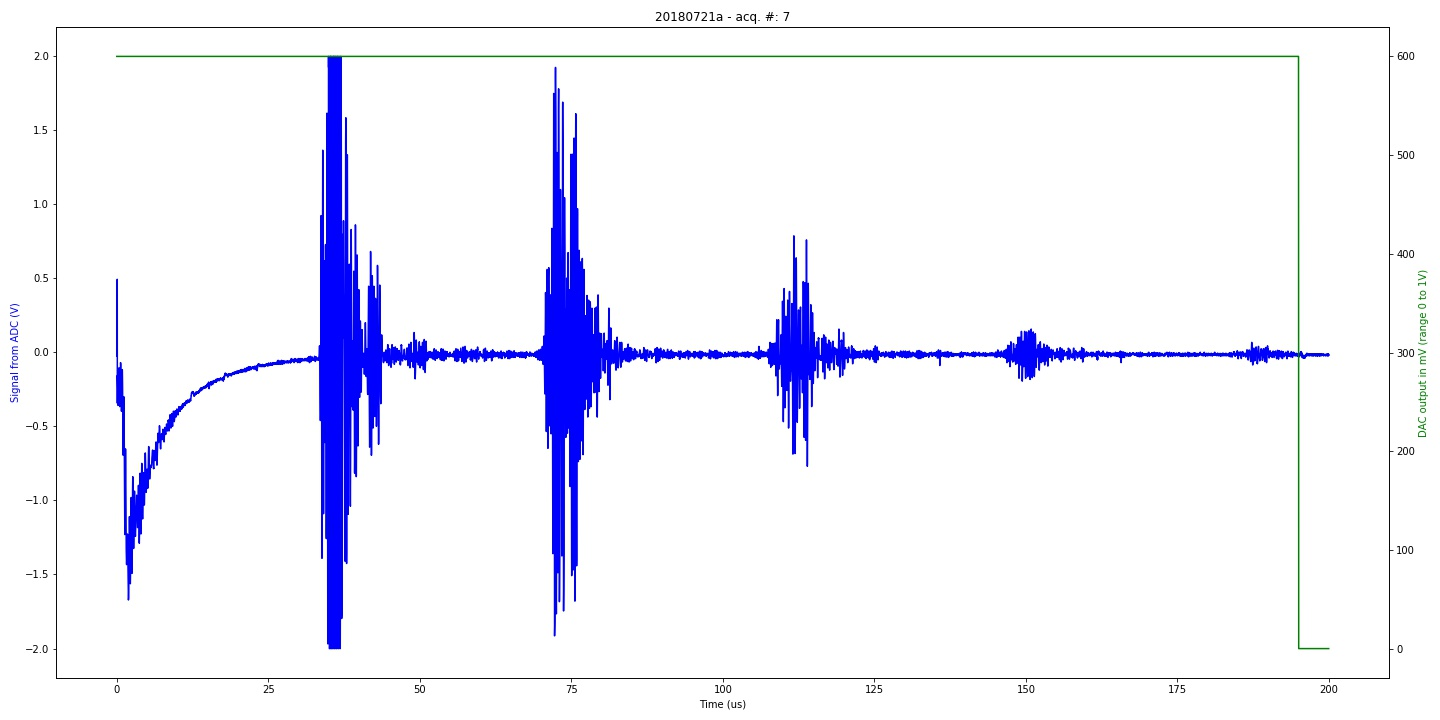Graph of 20180721a experiment. Classical experiment with calibration piezo (category: graph).Graph of 20180721a experiment. Classical experiment with calibration piezo (category: graph).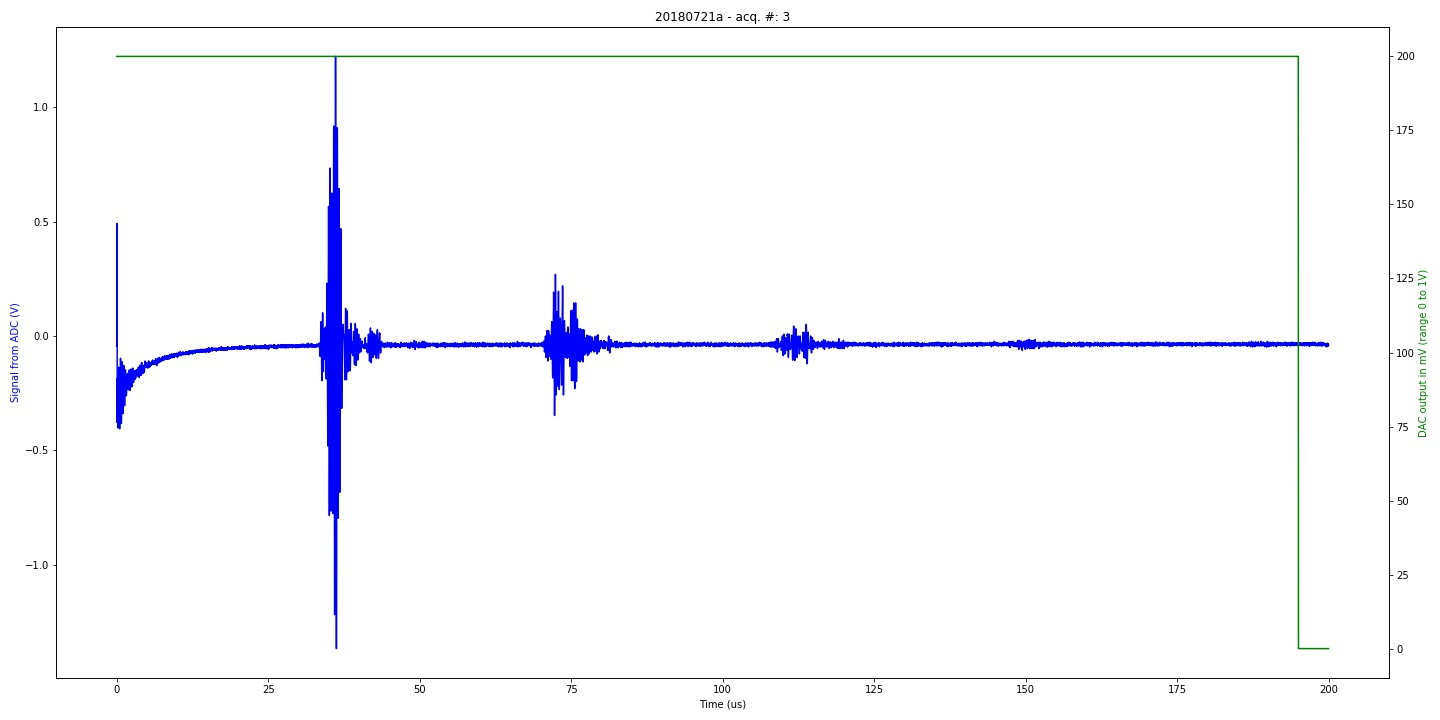Graph of 20180721a experiment. Classical experiment with calibration piezo (category: graph).Finding the AD8831 gain control calibration. (category: calibration).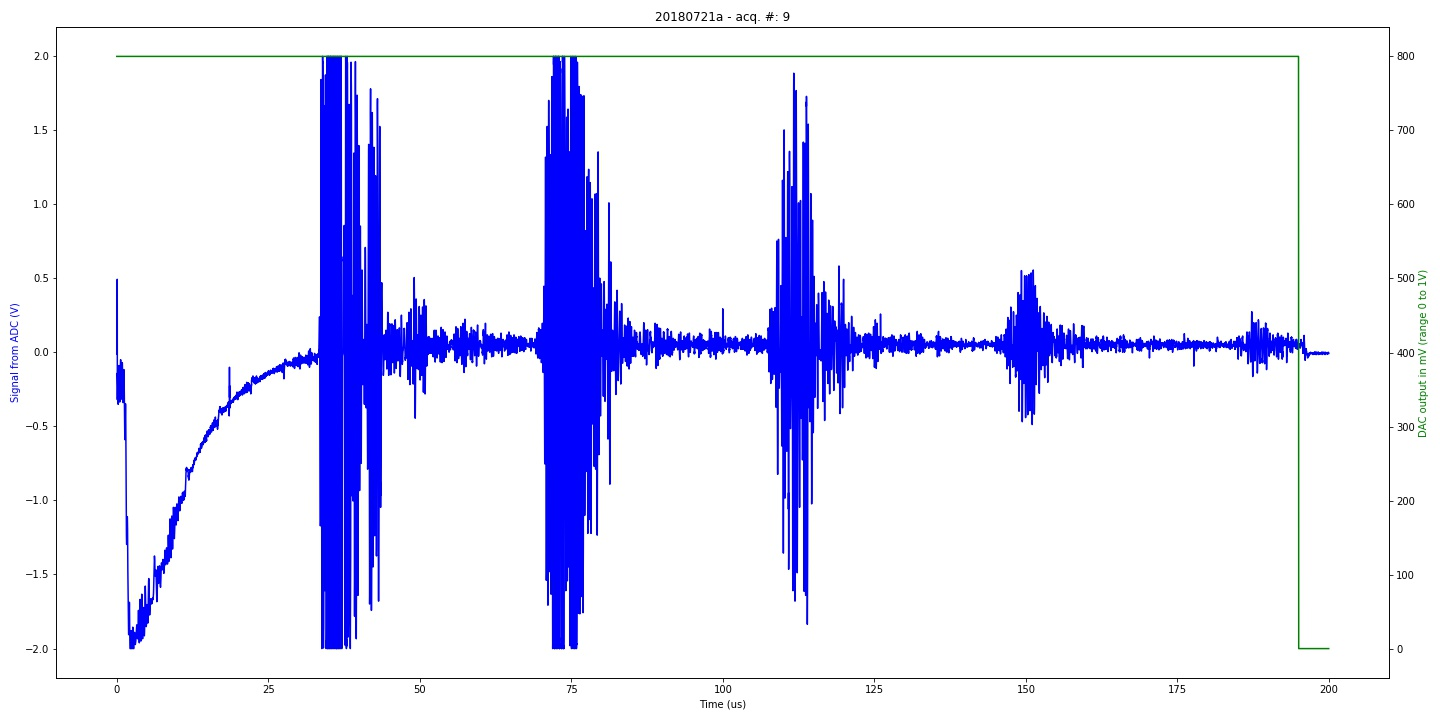Graph of 20180721a experiment. Classical experiment with calibration piezo (category: graph).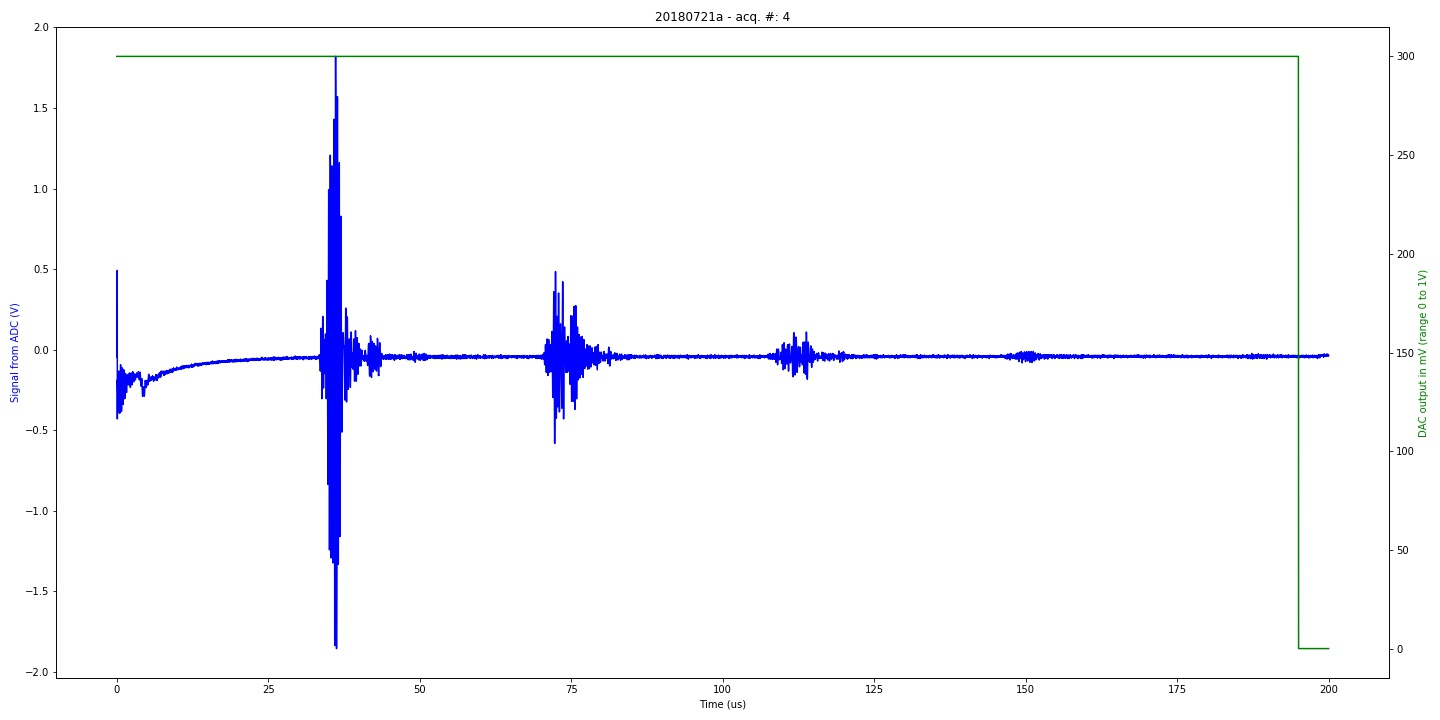Graph of 20180721a experiment. Classical experiment with calibration piezo (category: graph).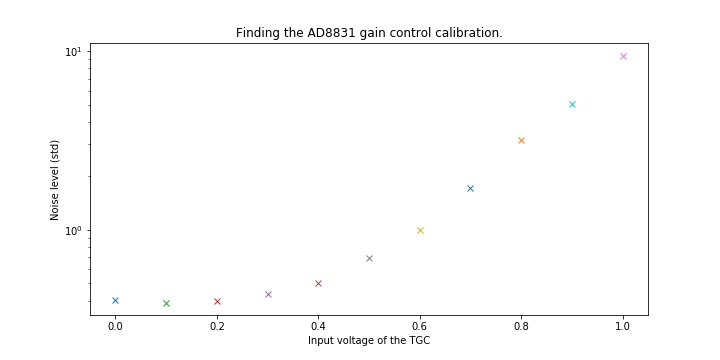Finding the AD8831 gain control calibration. (category: calibration).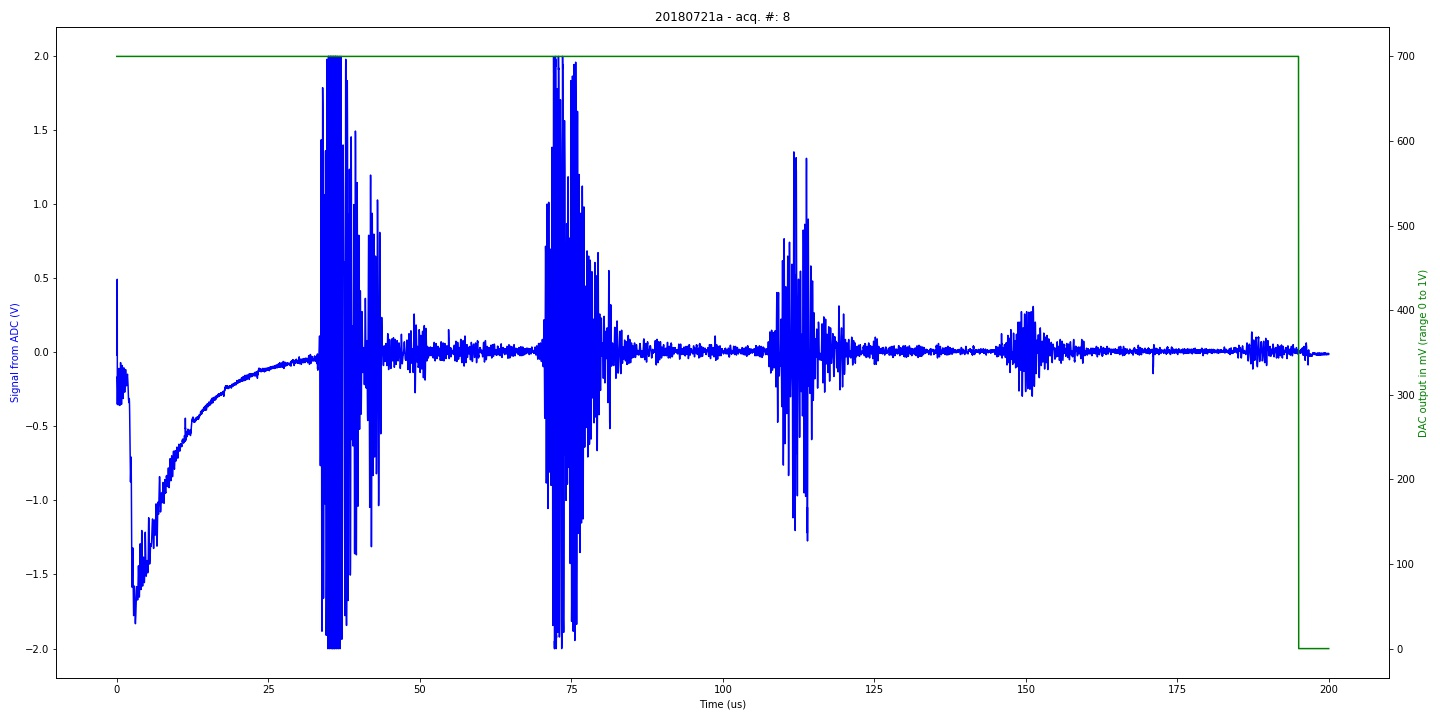Graph of 20180721a experiment. Classical experiment with calibration piezo (category: graph).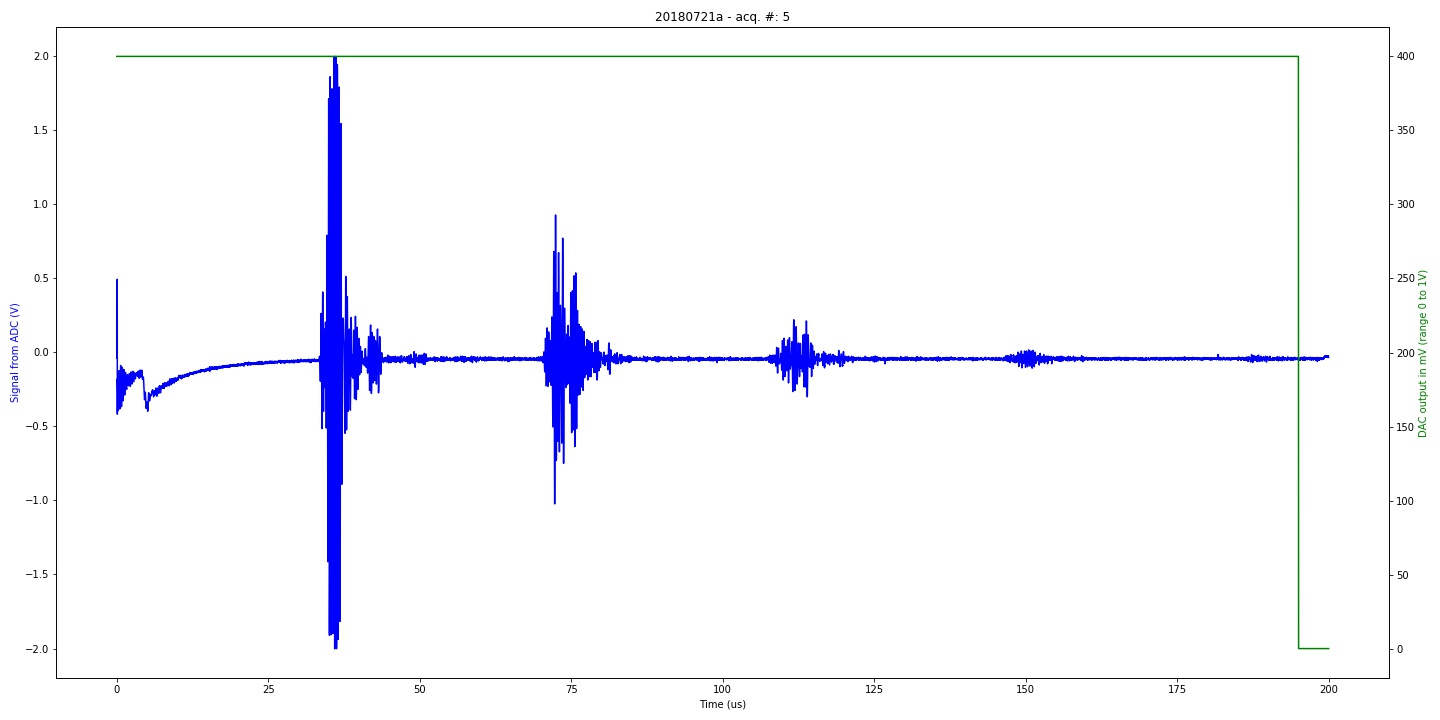Graph of 20180721a experiment. Classical experiment with calibration piezo (category: graph).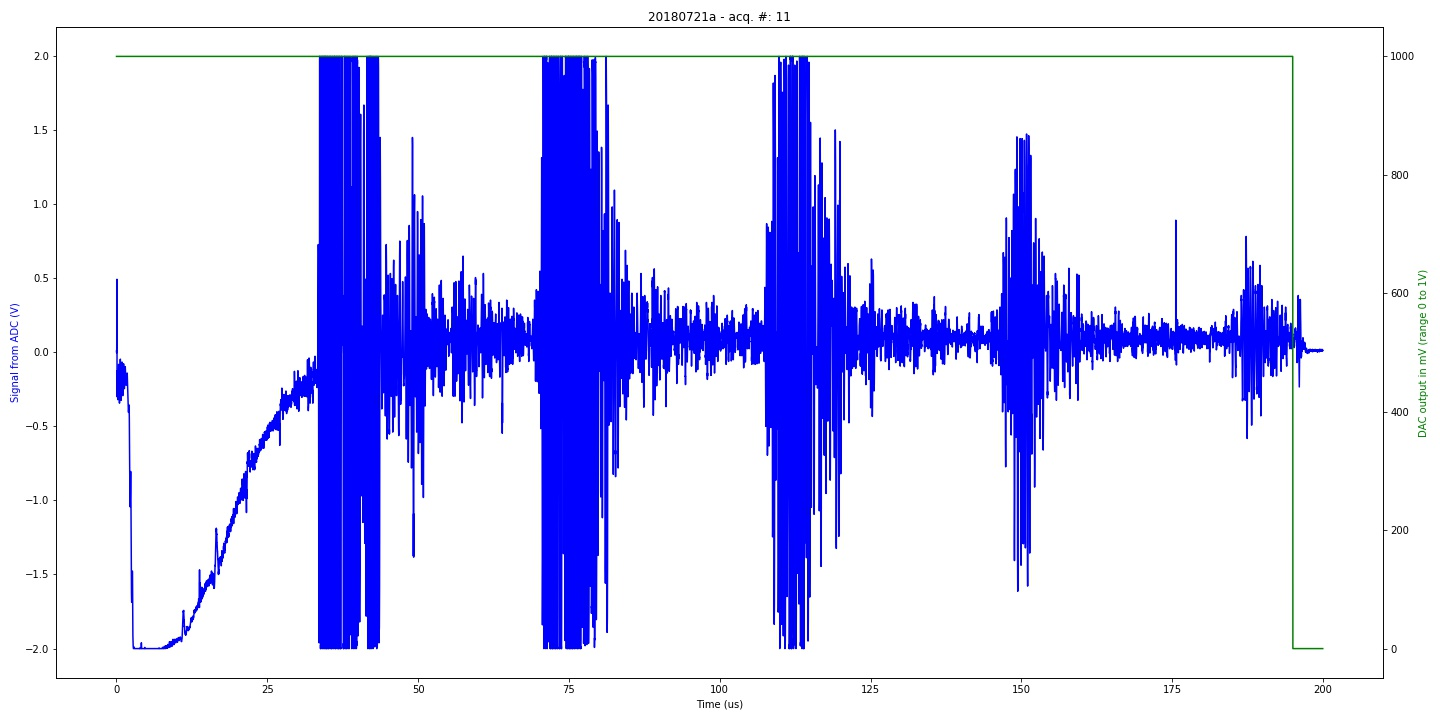Graph of 20180721a experiment. Classical experiment with calibration piezo (category: graph).Finding the AD8831 gain control calibration. (category: calibration).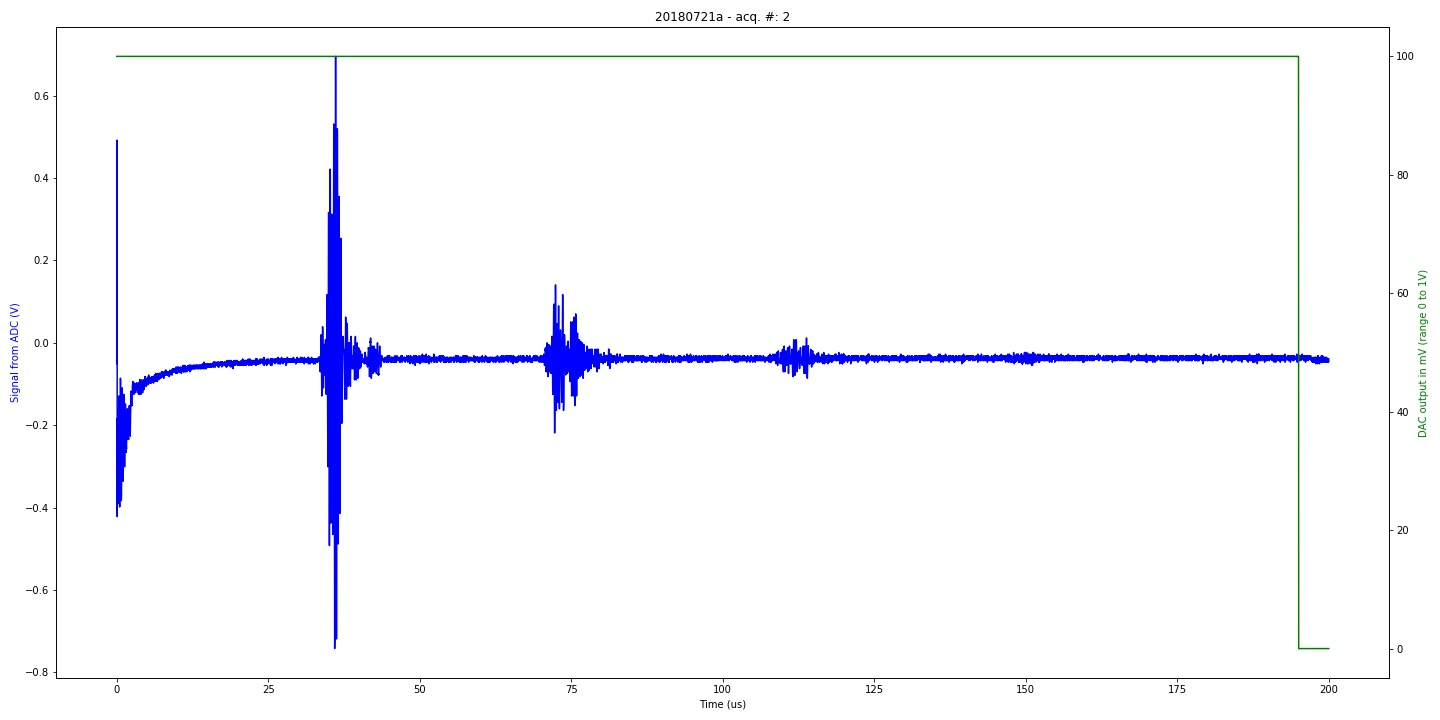Graph of 20180721a experiment. Classical experiment with calibration piezo (category: graph).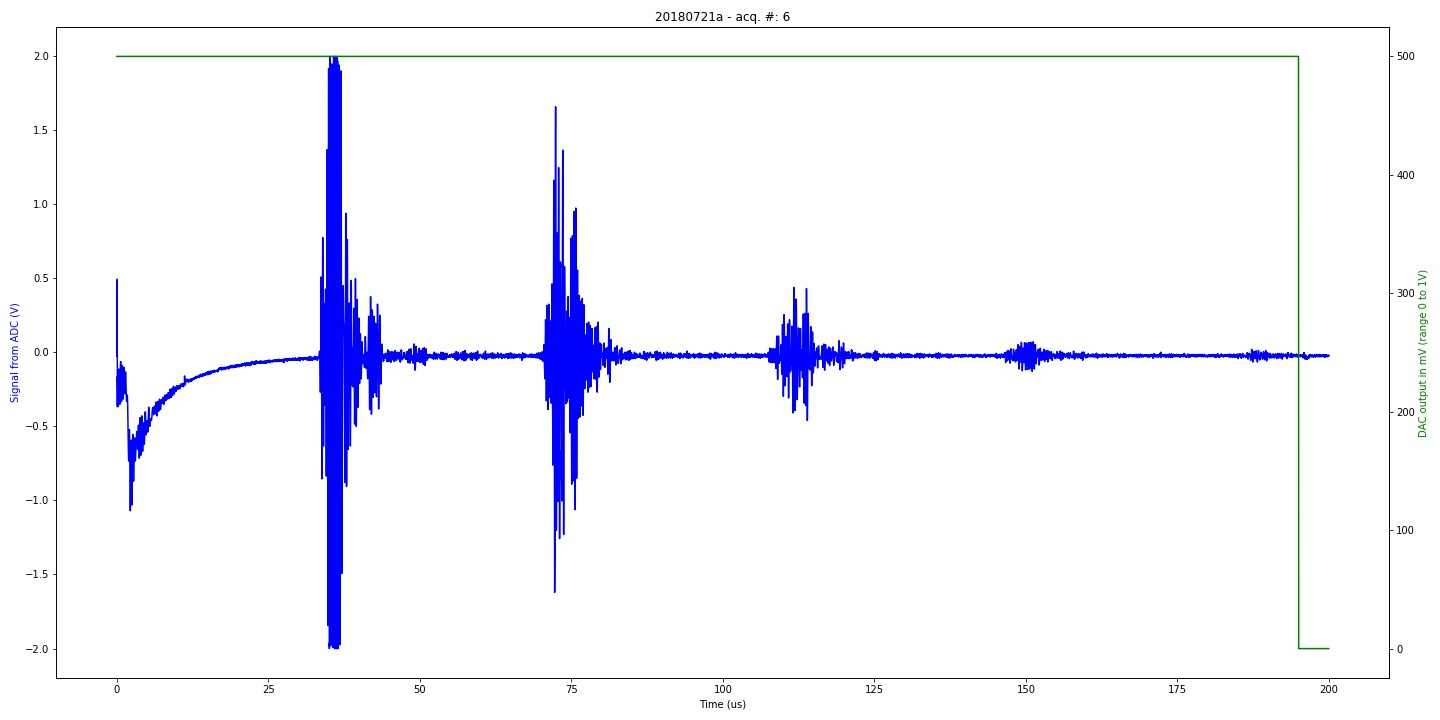Graph of 20180721a experiment. Classical experiment with calibration piezo (category: graph).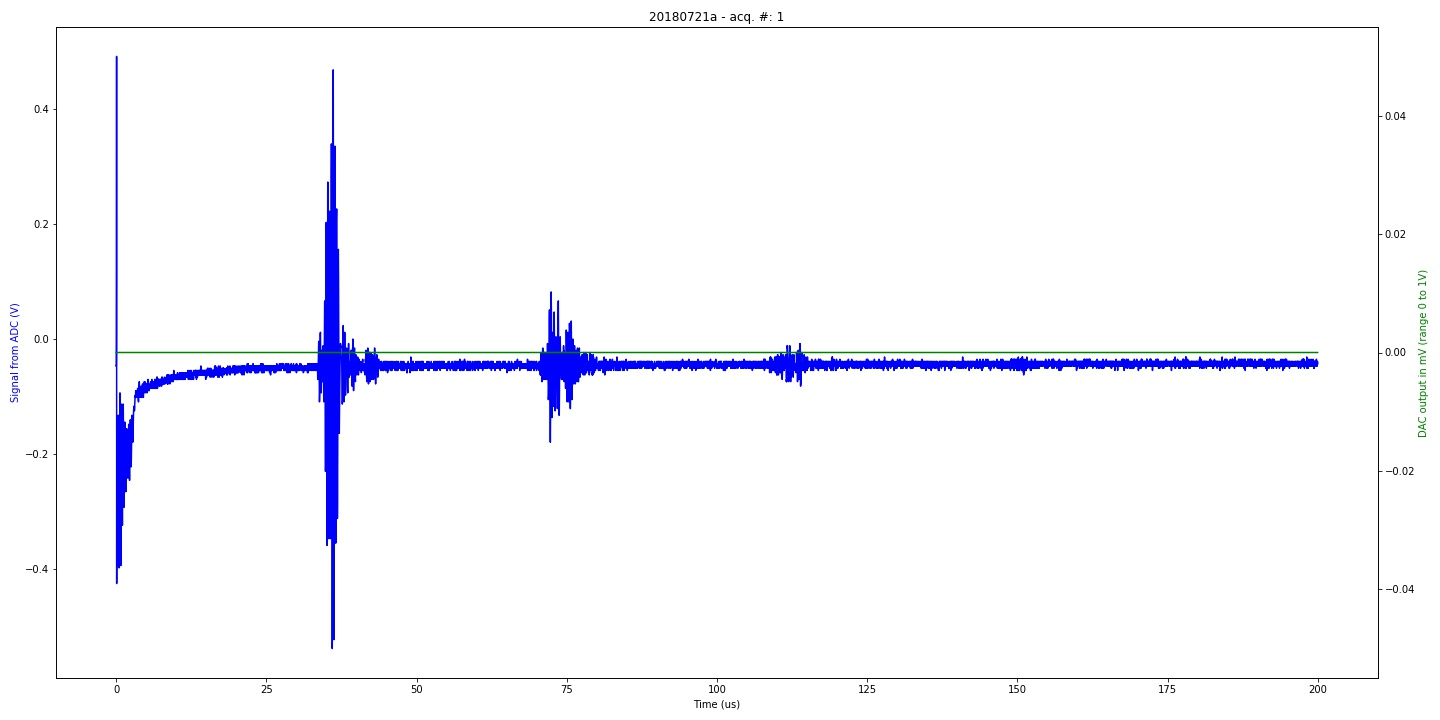Graph of 20180721a experiment. Classical experiment with calibration piezo (category: graph).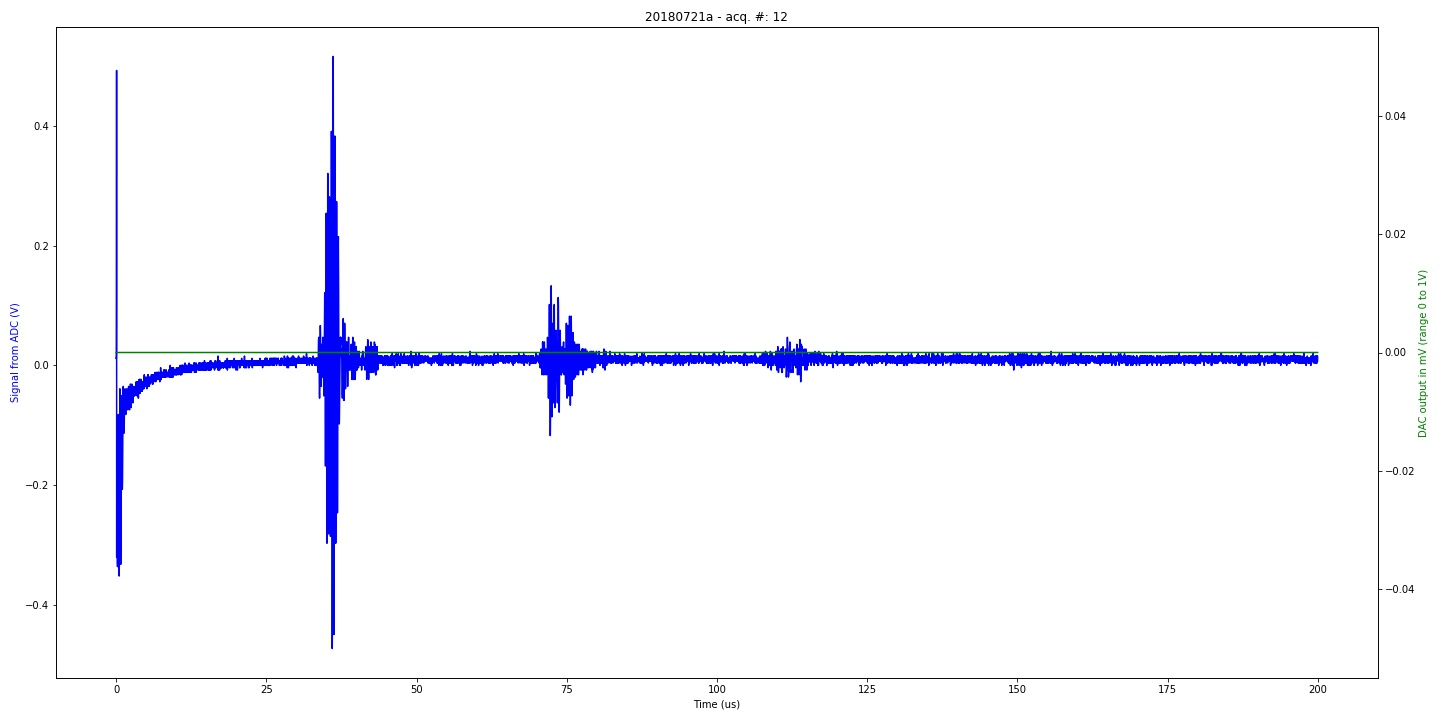Graph of 20180721a experiment. Classical experiment with calibration piezo (category: graph).